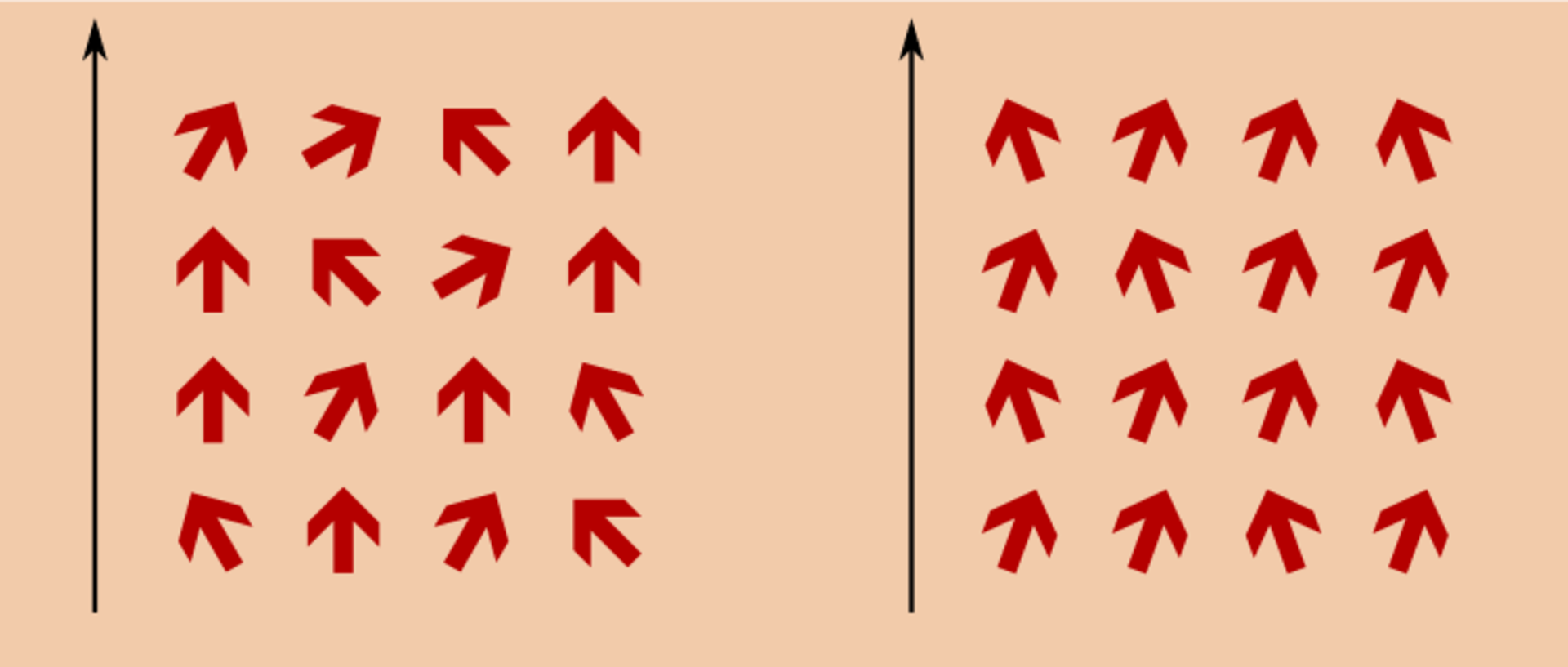# A PDE approach to mean-field systems

The main objective of statistical mechanics is to understand the large-scale behaviour of systems that are composed of very many individual components, such as gases, liquids, or crystalline solids, that interacts through locally simple rules. The statistical mechanics of disordered systems is a particularly difficult, but also particularly exciting, branch of the general subject, that is devoted to the same problem in situations when the interactions between these components are very irregular and inhomogeneous, and can only be described in terms of their statistical properties.

In the lecture we will focus on so-called (disordered) mean-field spin systems that are characterised by the fact that each individual component can interact with any other individual component.  One of the simplest model within this class is the Curie-Weiss model. Along the way, we will introduce analytical techniques related to the study of Hamilton-Jacobi equations, and use them to identify the limit of the so-called free energy free energy of this model.  Further, this strategy we be applied to a first class of disordered models coming from statistical inference. In terms of difficulty, these models are a useful bridge between the Curie-Weiss model and disordered mean-field spin systems. We will finally turn our attention to the latter models, in which infinite-dimensional Hamilton-Jacobi equations arise.

• #### Team

Lecturer: Martin Slowik

• #### Information

Vorbesprechung: Erste Semesterwoche

Ilias: Please register to the lecture on portal2 and/or Ilias

Content of the lecture:

• some basics of the theory of large deviations (level 1) and convex analysis
• Curie-Weiss model
• Hamilton-Jacobi equations
• the generalized Curie-Weiss model via Hamilton-Jacobi equations
• statistical interference
• some aspects of spin glasses